# DC Pandey Solutions: Work, Energy & Power - 1 Notes | Study DC Pandey Solutions for JEE Physics - JEE

## JEE: DC Pandey Solutions: Work, Energy & Power - 1 Notes | Study DC Pandey Solutions for JEE Physics - JEE

The document DC Pandey Solutions: Work, Energy & Power - 1 Notes | Study DC Pandey Solutions for JEE Physics - JEE is a part of the JEE Course DC Pandey Solutions for JEE Physics.
All you need of JEE at this link: JEE

Introductory Exercise 6.1

Q.1. A block is pulled a distance x along a rough horizontal table by a horizontal string. If the tension in the string is T, the weight of the block is W, the normal reaction is N and frictional force is F. Write down expressions for the work done by each of these forces.
Ans.
7x, O, O, - Fx

Work done by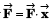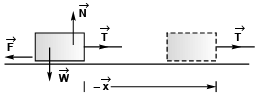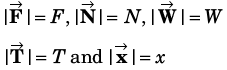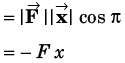Work done by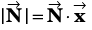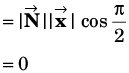Work done by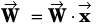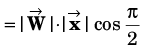Work done by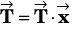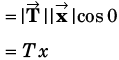Q.2. A particle is pulled a distance l up a rough plane inclined at an angle α to the horizontal by a string inclined at an angle β to the plane (α + β < 90°). If the tension in the string is T, the normal reaction between the particle and the plane is N, the frictional force is F and the weight of the particle is W. Write down expressions for the work done by each of these forces.
Ans.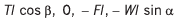Work done by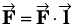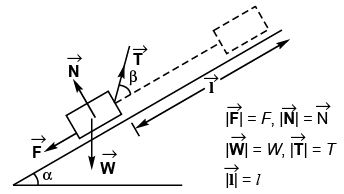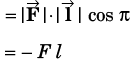Work done by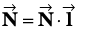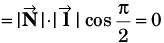Work done by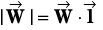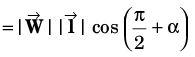= - Wl sin α
Work done by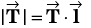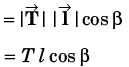Q.3. A bucket tied to a string is lowered at a constant acceleration of g/4. If the mass of the bucket is m and is lowered by a distance l then find the work done by the string on the bucket.
Ans: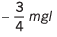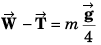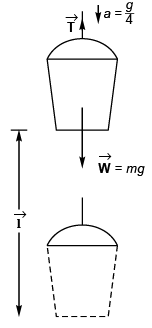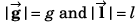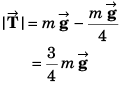Work done by string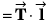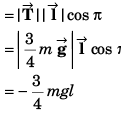Q.4. A 1.8 kg block is moved at constant speed over a surface for which coefficient of friction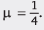It is pulled by a force F acting at 45° with horizontal as shown in figure. The block is displaced by 2 m. Find the work done on the block by (a) the force F (b) friction (c) gravity.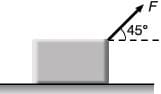Ans.
(a) 7.2 j  (b) - 7.2 J (c) zero

μN = F cos 45°              …(i)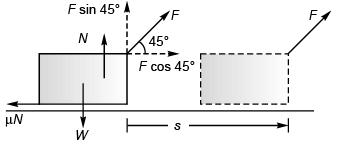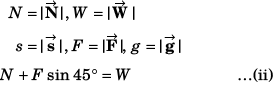Substituting value of N from Eq. (ii) in Eq. (i).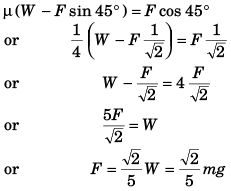Work done by force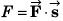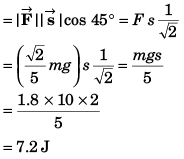Work done by friction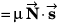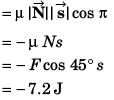Work done by gravity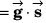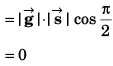Q.5. A small block of mass 1 kg is kept on a rough inclined wedge of inclination 45° fixed in an elevator. The elevator goes up with a uniform velocity v = 2 m/s and the block does not slide on the wedge. Find the work done by the force of friction on the block in 1 s. (g = 10 m/s2)

Ans 10 J

F = mg sin 45°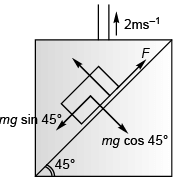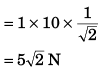Displacement of lift in 1s = 2 m
Work done  by force of friction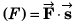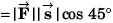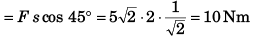Q.6. Two equal masses are attached to the two ends of a spring of force constant k. The masses are pulled out symmetrically to stretch the spring by a length 2x0 over its natural length. Find the work done by the spring on each mass.
Ans.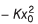Total work-done by spring on both masses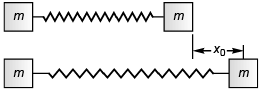= PE of the spring when stretched by 2x0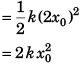∴  Work done by spring on each mass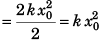Q.7. Force acting on a particle varies with displacement as shown in figure. Find the work done by this force on the particle from x = - 4 m t o x = + 4 m .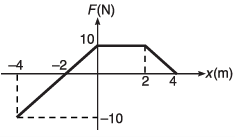Ans:
30J

Work done = Area under the curve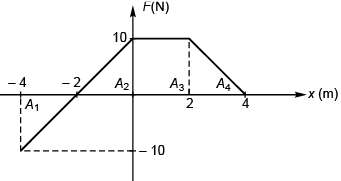= A+ A2 + A3 + A4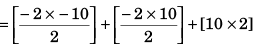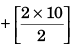= 30 Nm

The document DC Pandey Solutions: Work, Energy & Power - 1 Notes | Study DC Pandey Solutions for JEE Physics - JEE is a part of the JEE Course DC Pandey Solutions for JEE Physics.
All you need of JEE at this link: JEEUse Code STAYHOME200 and get INR 200 additional OFF

## DC Pandey Solutions for JEE Physics

210 docs

Track your progress, build streaks, highlight & save important lessons and more!

,

,

,

,

,

,

,

,

,

,

,

,

,

,

,

,

,

,

,

,

,

,

,

,

;